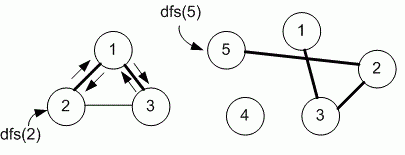Competitions

# Depth First Search

Undirected graph is given. Run depth first search from the given vertex v and print the numbers of vertices in the order of their first visit.

#### Input

First line contains number of vertices n (n100) and edges m of undirected graph. Each of the next m lines contains two vertices a and b - an undirected edge of the graph. The last line contains vertex v.

#### Output

Run dfs(v) and print in one line the numbers of vertices in the order of their first visit.Time limit 1 second
Memory limit 128 MiB
Input example #1
3 3
1 2
2 3
1 3
2

Output example #1
2 1 3

Input example #2
5 3
1 3
2 3
2 5
5

Output example #2
5 2 3 1